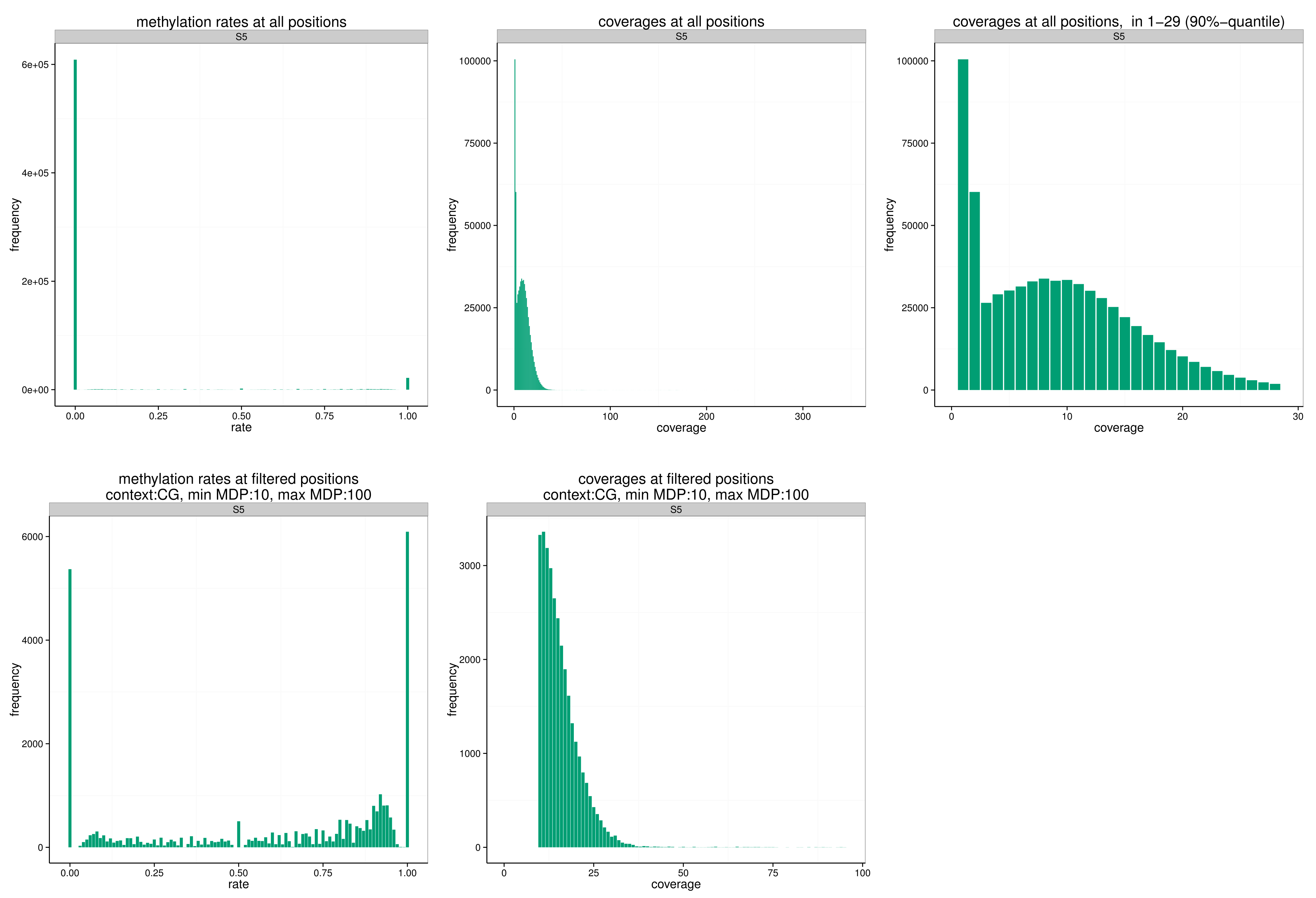# Example: Calling¶

## Example: BAT_calling ¶

(USAGE: BAT_calling)

### Command¶

``````BAT_calling -d genomes/hg19/hg19_chr19.fa -q mapped/S5.bam -o called/
``````

### Output files¶

``````called/S5.vcf.gz
called/S5.calling.log
``````

### View¶

``````\$ zcat called/S5.vcf.gz | head -20
##fileformat=VCFv4.1
##reference=file:///genomes/hg19/hg19_chr19.fa
##contig=<ID=chr19,length=59128983>
##INFO=<ID=CS,Number=1,Type=Character,Description="Bisulfite conversion strand, i.e., strand of cytosine relative to reference">
##INFO=<ID=CC,Number=1,Type=String,Description="Sequence context of two bases on the reference relative to bisulfite conversion strand, missing value if context is beyond reference boundaries">
##INFO=<ID=NS,Number=1,Type=Integer,Description="Number of samples with data">
##INFO=<ID=MMR,Number=1,Type=Float,Description="Mean methylation rate among samples with data">
##INFO=<ID=DMR,Number=1,Type=Float,Description="Difference in methylation rate (Sample1 - Sample2).">
##FORMAT=<ID=DP,Number=1,Type=Integer,Description="Total Depth, not filtered by mapping quality, base quality, or bisulfite conversion information">
##FORMAT=<ID=MDP,Number=1,Type=Integer,Description="Number of reads after filtering by bisulfite conversion strand">
##FORMAT=<ID=MDP3,Number=3,Type=Integer,Description="Number of reads with 1) unconverted cytosines, 2) converted cytosines or thymines, and 3) other bases after filtering by bisulfite conversion strand">
##FORMAT=<ID=MRDP,Number=1,Type=Integer,Description="Number of reads with unconverted (methylated) and converted (unmethylated) cytosines after filtering by bisulfite conversion strand">
##FORMAT=<ID=CM,Number=1,Type=Integer,Description="Number of reads with unconverted (methylated) cytosines after filtering by bisulfite conversion strand">
##FORMAT=<ID=CU,Number=1,Type=Integer,Description="Number of reads with converted (unmethylated) cytosines after filtering by bisulfite conversion strand">
##FORMAT=<ID=MR,Number=1,Type=Float,Description="Estimated methylation rate">
#CHROM  POS     ID      REF     ALT     QUAL    FILTER  INFO    FORMAT  S5
chr19   117927  .       C       .       .       .       CS=+;CC=CA;NS=1;MMR=1.00;DMR=.  DP:MDP:MDP3:MRDP:CM:CU:MR       1:1:1,0,0:1:1:0:1.00
chr19   117932  .       C       .       .       .       CS=+;CC=CT;NS=1;MMR=0.00;DMR=.  DP:MDP:MDP3:MRDP:CM:CU:MR       1:1:0,1,0:1:0:1:0.00
chr19   117935  .       C       .       .       .       CS=+;CC=CA;NS=1;MMR=0.00;DMR=.  DP:MDP:MDP3:MRDP:CM:CU:MR       1:1:0,1,0:1:0:1:0.00
chr19   117941  .       C       .       .       .       CS=+;CC=CC;NS=1;MMR=0.00;DMR=.  DP:MDP:MDP3:MRDP:CM:CU:MR       1:1:0,1,0:1:0:1:0.00
``````

(top)

## Example: BAT_filter_vcf ¶

(USAGE BAT_filter_vcf)

### Commands¶

``````\$ for i in called/S[1-8].vcf.gz; do
o=`echo \$i | sed 's/.vcf.gz/_CG.vcf.gz/'`;
BAT_filter_vcf --vcf \$i --out \$o --context CG --MDP_min 10 --MDP_max 100;
done
``````

### Output files¶

``````...
called/S5_CG.vcf.gz
called/S5_CG.bedgraph
called/S5_CG.pdf
...
``````

### View¶

``````\$ head -3 called/S5_CG.bedgraph
chr19   4514953 4514954 0.60
chr19   9294488 9294489 0.93
chr19   9294489 9294490 0.79
``````
``````called/S5_CG.pdf
``````(top)